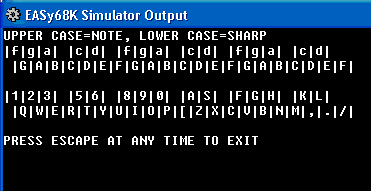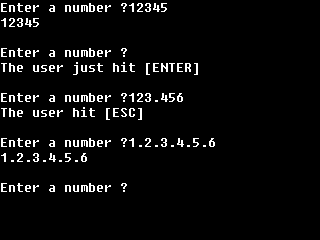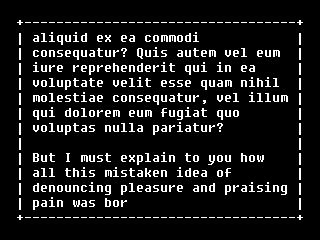Random by Lee Davison: generates a binary file of 65536 pseudo random bytes which can be analyzed to gauge the effectiveness of the random number generator. This site made possible in part by adds like this. Please show your support for EASy68K by visiting their sites.Fast Floating Point Calculator for the 68000: by Paul Santa-Maria     CALC operations:  + add, - subtract, negate, * multiply, ** power, / divide,     ? compare, \$ specify floating point number in hexadecimal,     ( parenthesis, SQRT( square root, SIN( sine, COS( cosine,     TAN( tangent, EXP( exponent, LOG( logorithm, ATAN( arctangent     SINH( hyperbolic sine, COSH( hyperbolic cosine, TANH( hyperbolic tangent     TST( test, NEG( negate, ABS( absolute value, INT( integer The calculator will flag overflow, divide by zero, and negative square root errors. Keyboard by Emerson Williams: A musical keyboard Structured Syntax Example - Determines if the user input number is prime. Used as an example of structured assembly. String Macros by Benjamin Collins: String macros included are: length, at, compare, erase, find, replace and insert. Basic Calculator by Paul Jay: Basic arithmetic with variable storage. Accepts input in the form [operand1] [operator] [operand2].Manometer by Christopher Cesarz: Calculates pressure difference of a manometer Getstring by Lee Davison: is a small function to return a string consisting of only user defined valid characters. Any invalid characters are ignored and not echoed to the screen.And now a word from one of our sponsors. Scroll demo by Lee Davison: An example of using the scroll text rectangle task.

Last Updated December 11, 2013